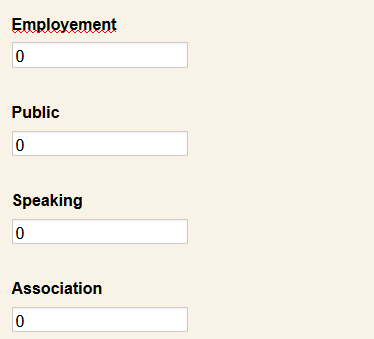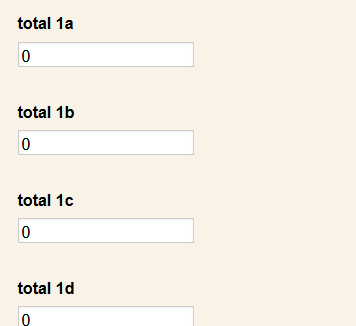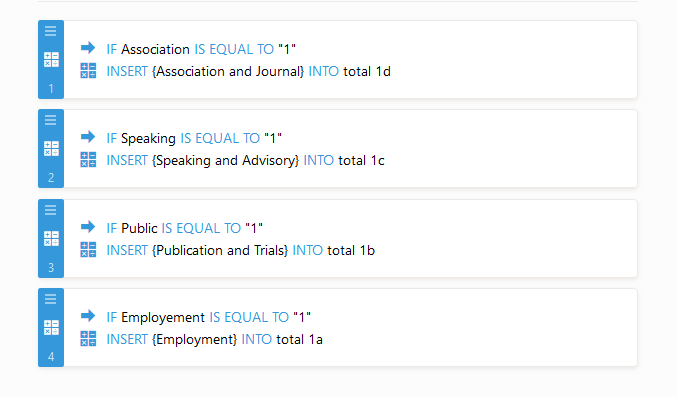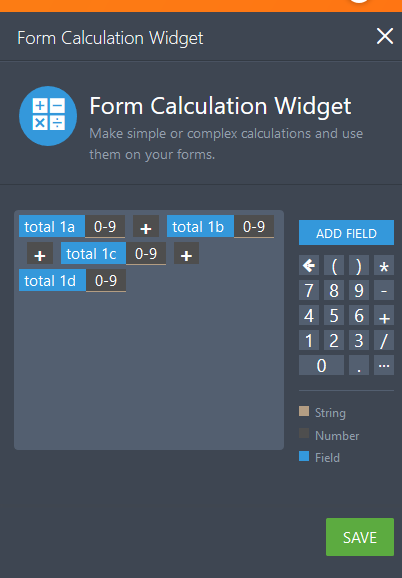# How to get values of 1,2 and 3 from the caluclation ?

•erinvistnes
Asked on July 09, 2019 at 03:51 PM
I now have a different question regarding the score for my four single-choice questions. I would like to count the number of 1's, 2's, and 3's that are scored for the set of four questions. To do so, I have created an array in the form calculation titled "HCP Score Array." Next, I would like to calculate the number of 1's, 2's and 3's in that HCP Score Array, but I am unsure how to do that. The "count" function in the Form Calculator seems to only count the number of responses to a question, rather than the value scored in that question. Any advice?
•Richie_P
Answered on July 09, 2019 at 04:32 PM

Unfortunately, there is no formula to determine the total of your array.

We can get the number of values selected using the visual report.

However, if you wish to calculate the total using your form, you would have to separate container for values with answer of 1 by using conditions.

Example:

1)We create 4 Form Calculation fields to hold the values for each question.2) We add another 4 field to hold the value if the answer is 1and we will use condition to check the value.You would need to repeat this steps for values 2 and 3.

3) Add a field to calculate the total. You would need to add separate  fields for total values 1, 2 and 3.Here is my sample form:https://form.jotform.com/91895756839986

This will get the total values of 1 if selected.

Please give it a try and let us know if you need further assistance.